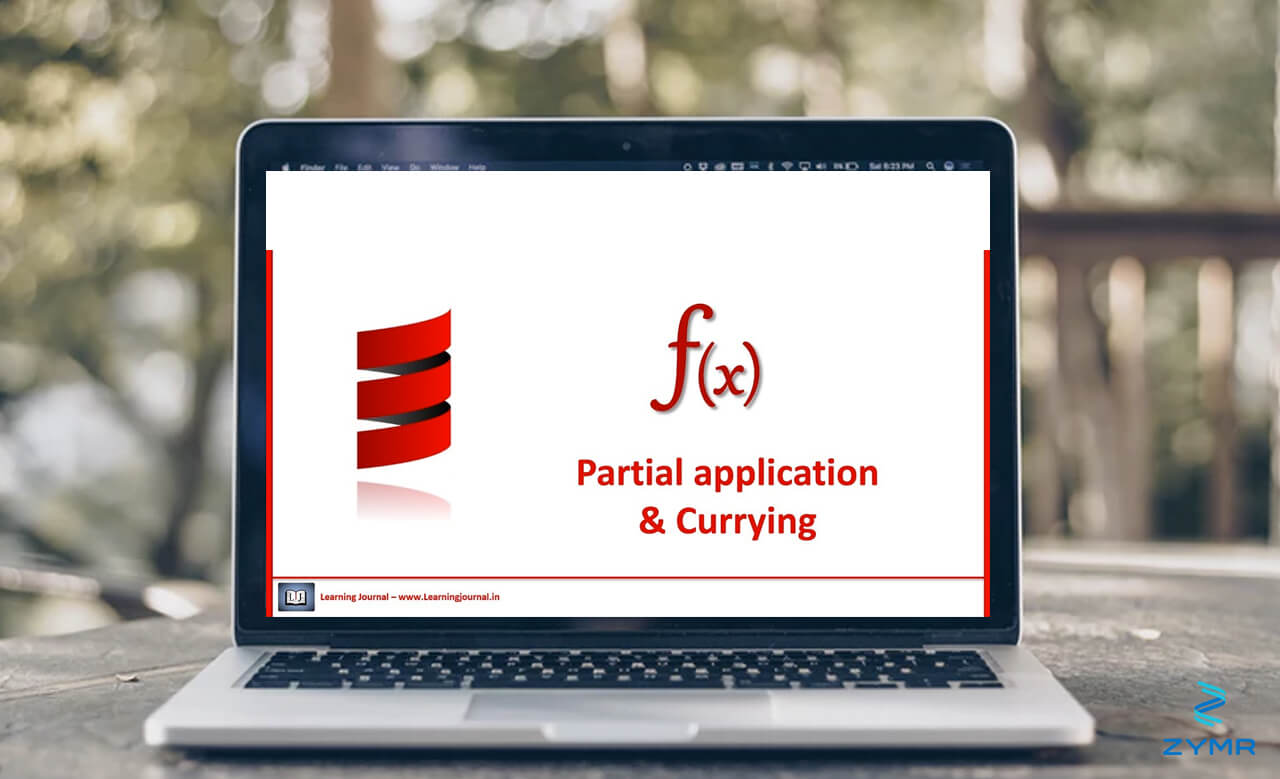Here we are going to take a look at Scala Partially Applied, Partially Defined, Anonymous, and Curried Functions.

Twitter wrote much of its website in Scala. They have written this style guide and reference material that is recommended to study.

### Partially Applied Functions

Do not confused Partially Applied Functions with Partially Define Functions (defined below). A Partially Applied function is one where you send it fewer than the required number or arguments.

For example, first define a function square() that takes a double and input and returns a double:

def square(d: Double) : Double = { return (d*d) }

Then take a function that multiplies the two input parameters:

def multiply(x : Double, y: Double): Double = { return(x * y)}

Then nest them together to multiply the first number times the second, but first square the second:

var d = multiply(10,square(4))

### Partially Defined Functions

This is a unary function that works only for certain values. (Unary means it accepts only one argument.) This idea maps perfectly to the mathematical concept of a domain. For example, the natural logarithm is not defined for any x < =0. We can express that in Scala. Define a function l and use the case statement to limit the parameters it will accept: import scala.math._ val l: PartialFunction[Double, Double] = { case x if x > 0 => log(x)}

Then ask what the natural logarithm of the constant e is:

l(E)

Double = 1.0

You can test whether a partial function is defined for a particular value by using isDefinedAt()

l.isDefinedAt(0)

Returns:
Boolean = false

### Anonymous Function

This just means an inline function.

val double = (x: Int) => x * 2
double(2)

Returns:
Int = 4

### Function Currying

To curry means to take a function that requires multiple arguments into > 1 functions that take a single argument.

So define a function to multiply two numbers:

def multiply(x: Int, y: Int) = x * y

Then curry it like this:

def multiply(x: Int) = (y:Int) => x * y

Now call it like this:

multiply(2)(3)